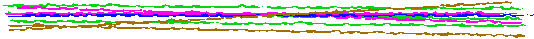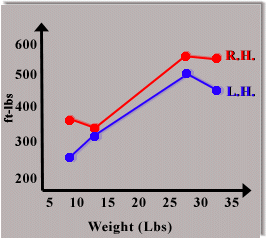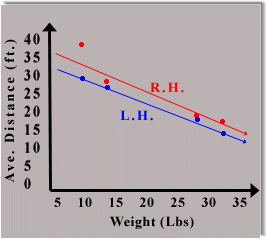The Relationship between
Throwing Distance and Object WeightPurpose:  This week, Scientific AmeriKen will investigate the relationship between the distance an object is thrown and the weight of the object. An investigation sure to have powerful real world applications.Hypothesis:  It is the hypothesis of this experiment is a linear relationship exist between throwing distance and the weight of an object. This implies that distance will have an inverse relationship with weight such that an increase in weight will decrease distance and vice-versa. This also implies that theoretical maximum distances and weights can be found for the thrower.Equipment:  Needed for this experiment was a scale, rocks of various sizes, a pen and paper.Procedure:  The first step is to measure and record the weight of the rocks, then throw the rocks in a consistent manner. Rocks thrown in this experiment were done so in a "two handed shot put" style.  Pace off or measure with a measuring tape the distance the rock travels. For each rock, repeat twice for right handed throws and twice for left handed throws. Average out the total from each trial, and plot weight vs  ave. distance traveled. Also, for each trial multiply distance traveled by the weight and compare for all trials.Results:

Right Handed Results
 Weight (lbs.) Trial #1 Distance (ft.) Trial #2 Distance (ft.) Ave Distance (ft.) Distance x Weight (ft. x  lbs) 9.5 Pounds Right Hand 37 Feet 37 Feet 37 Feet 351.5 ft-lbs 12.5 Pounds Right Hand 28 Feet 27 Feet 27.5 Feet 343.8 ft-lbs 27 Pounds Right Hand 19.5 Feet 21.5 Feet 20.5 Feet 553.5 ft-lbs 32 Pounds Right Hand 17 Feet 17.5 Feet 17.25 Feet 552.0 ft-lbs

Left Handed Results
 Weight (lbs.) Trial #1 Distance (ft.) Trial #2 Distance (ft.) Ave Distance (ft.) Distance x Weight (ft. x  lbs) 9.5 Pounds Left Hand 29 Feet 27 Feet 28 Feet 266.0 ft-lbs 12.5 Pounds Left Hand 26 Feet 25 Feet 25.5 Feet 318.8 ft-lbs 27 Pounds Left Hand 18 Feet 19 Feet 18.5 Feet 499.5 ft-lbs 32 Pounds Left Hand 14.5 Feet 14 Feet 14.25 Feet 456.0 ft-lbs

 Graph 1: Illustrates amount of force applied as a function of the weight of the rock.  R.H.= Right handed throws L.H.= Left handed throwsGraph 2: Illustrates Distance rocks traveled as a function of their weight.  Eq of R.H. Throws R.H. : Y= -.698X + 39.1 Yint=39.1 (Theoretical Max Dis. any rock can be thrown in feet) Xint=56.1 (Theoretical heaviest Rock that can be tossed in lbs) Eq of L.H. Throws L.H. : Y= -.645X + 34.5 Yint=34.5 (Theo. Max Dis) Xint=53.5 (Theo. Max Weight) For how this information was found click hereConclusion:  The results of this experiment yield some interesting information. Limited data allow for only a subjective view of the hypothesis's correctness. Judging from graph #2 it would seem as though linear best fit lines work nicely for L.H. results and moderatly well for R.H. results. This implies that the relationship between weight and throwing distance is linear. However, if this is the case, it would imply that a rock of greater weight then 56.1 lbs could not be thrown using the "shot putt" style, a fact that will remain untested until a rock of such size is found. Another interesting result which does not support the hypothesis is the large difference in amount of ft-lbs in the different throws. It is noted how the amount of force applied by the thrower increases as the weight of the object increases. Most likely one would expect that if distance had a linear relationship with object weight, then one would expect that inputed force would remain constant for all throws so that the only two variables remain distance or weight. For the sake of argument, Scientific AmeriKen accepts this second explanation, thus refuting the hypothesis, as we hope that we should be able to throw a wussy little 60 pound rock.